Next: Application to Generalized XOR Up: Lower Oracle Query Bounds Previous: The Quantum Adversary:   Contents

# A Lemma for Proving Lower Query Bounds

The sensitivity of an input x of a function f is the number of Hamming neighbors y of x such that f (x)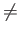f (y). We will use the following lemma to establish lower bounds for functions based solely on their sensitivity for a particular input.

Lemma 2.2.1   Let f be an N-bit Boolean function. If some input x has k Hamming neighbors y such that f (x)f (y), then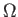(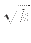) oracle queries are required to compute f in the bounded error setting.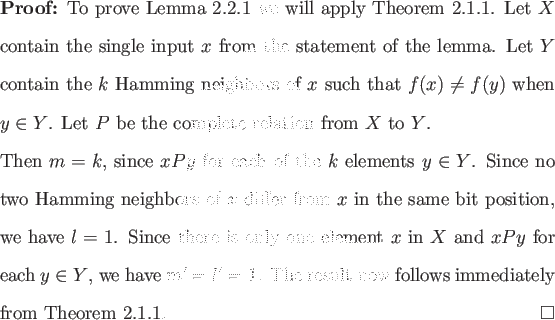This lemma will not in general provide us with the best lower bound that can be attained from Ambainis' Theorem 2.1.1: we are maximizing m/l, but we make no attempt to maximize m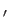/l. The strongest results of Ambainis' Theorem frequently arise from maximizing both. We will maximize both when dealing with partially symmetric functions in Theorem 2.5.1 and graph connectivity in Theorem 3.2.1. The singleton class of functions described in Sections 2.3 and 2.4 is a class for which Lemma 2.2.1 obtains optimal results.

SubsectionsNext: Application to Generalized XOR Up: Lower Oracle Query Bounds Previous: The Quantum Adversary:   Contents
Matthew Hayward Lower Query Bounds in the Quantum Oracle Model GitHub Repository